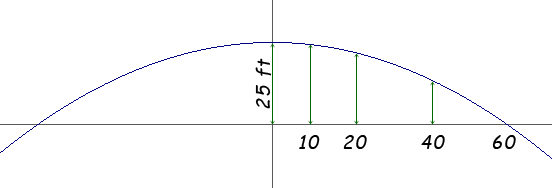SEARCH HOMEMath Central Quandaries & QueriesQuestion from Nisa, a student: A parabolic arch has a span of 120 feet and a maximum height of 25 feet. Choose suitable rectangular coordinate axes and find the equation of the parabola. Then calculate the height of the arch at points 10 feet,20feet,and 40 feet from the center.Hi Nisa.

Where are you stuck? I'll assume it is at the beginning, so I'll show you how to get started.

Since you can choose where the origin of your coordinate system will be, you want to make it convenient for yourself. Why not place the origin at the middle of the span? Then you can draw it thus:The equation of a parabola which opens down is y - yV= -A (x - xV)2, where (xV, yV) is the vertex (in your case, this is (0,25)) and A is a constant affecting the curvature. Thus your equation is just y = -Ax2 + 25.

Use one of the end-points of the arch such as (60, 0), to find the value of A. Then you have a suitable equation.

I chose this coordinate system because it makes it easy to find the height of the arch given the distance from the center. The distance from the center is just the x value and the height at that location is the y value.

So plug x = 10, 20, 40 into your equation and find your three corresponding heights.

Cheers,
Stephen La Rocque.Math Central is supported by the University of Regina and The Pacific Institute for the Mathematical Sciences.